## 1. OCR综述

### 1.1. 什么是OCR

OCR(Optical Character Recognition,光学字符识别)是指对图像进行分析识别处理，获取文字和版面信息的过程，是典型的计算机视觉任务，通常由文本检测和文本识别两个子任务构成。

• 文字检测：将图片中的文字区域位置检测出来（如图1(b)所示）；
• 文字识别：对文字区域中的文字进行识别（如图1(c)所示）。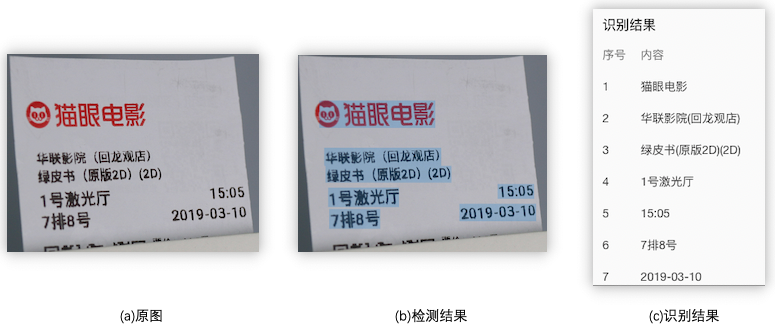### 1.2. OCR发展历程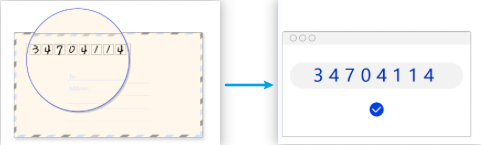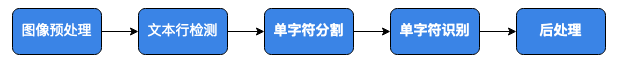• 图像预处理主要是对图像的成像问题进行修正，包括几何变换（透视、扭曲、旋转等），去模糊、光线矫正等；
• 文本检测通常使用连通域、滑动窗口两个方向；
• 字符识别算法主要包括图像分类、模版匹配等。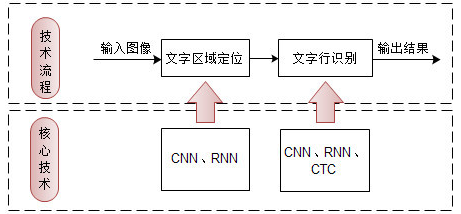### 1.3. OCR 常用检测方法

OCR文字检测就是将图片中的文字区域检测出来。

#### 1.3.1. 基于回归的方法

 - box回归

• 优点：对规则形状文本检测效果较好
• 缺点：无法准确检测不规则形状文本。

 - 像素值回归

Rosetta

CRNN

STAR-Net

RARE

SRN

### 1.5. OCR常用评估指标

 - 检测阶段：先按照检测框和标注框的IOU评估，IOU大于某个阈值判断为检测准确。这里检测框和标注框不同于一般的通用目标检测框，是采用多边形进行表示。

 - 识别阶段： 字符识别准确率，即正确识别的文本行占标注的文本行数量的比例，只有整行文本识别对才算正确识别。

 - 端到端统计：

### 1.6. 应用场景

• 通用场景：办公文档、广告图、表格、手写数字、自然场景图等；
• 卡证：身份证、银行卡、营业执照、名片等；
• 汽车：车牌、驾驶证、合格证等；
• 财务票据：火车票、飞机票、银行支票等；
• 医疗票据：医疗发票、病例首页等；
• ...

### 1.7. OCR面临的挑战## 2. OCR 检测方法

### 2.1. CTPN

#### 2.1.1. 模型介绍

CTPN（Connectionist Text Proposal Network，Detecting Text in Natural Image with Connectionist Text Proposal Network是目标检测算法Faster R-CNN 的改进算法，用于文字检测。CTPN根据文本区域的特点做了专门的优化：

• 使用更加符合自然场景文字检测特点的anchor（相比于物体，文字尺寸小）；
• 引入RNN用于处理场景文字检测中存在的序列特征；
• 引入Side-refinement(边界优化)提升文本框边界预测精度。

#### 2.1.2. 模型结构

CTPN采用的方法是将文本行分割成一个个小块（长度是固定的），然后去检测这些小块，最后使用一种文本行构造法将所有块连起来，如 图1 所示。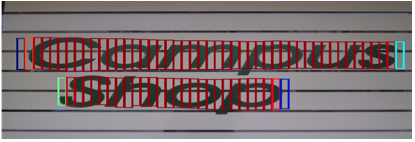CTPN网络结构如 图2 所示：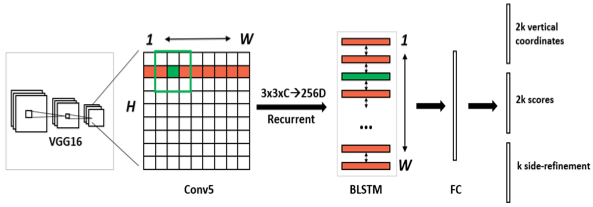- 准备数据集、并获取锚点anchor；

 - 输入图片，经过backbone（用VGG16的前5个Conv stage，即Conv5），提取图片feature map。经过VGG16之后得到的特征图长宽是原图的1/16，通道是512。

 - 在Conv5得到的feature map上再做卷积核大小为3，步长为1的卷积，进一步提取特征，用于预测当前卷积核所在位置k个anchor对应的类别信息、位置信息。其中，C表示通道数。

 - 把每一行的所有窗口对应的3*3*C的特征输入到BiLSTM（双向LSTM）网络中，提取文字的序列特征，这时候得到的特征是图像特征和序列特征的融合。

 - 将第三步得到的特征输入到FC全连接层，并将FC层特征输入两个分类或者回归层中。

CTPN任务1的输出是 $2k$ ，用于预测候选区域box的起始 $y$ 坐标和高度 $h$；任务2是用来对前景和背景两个任务的分类评分；任务3是 $k$ 个输出的side-refinement的偏移（offset）预测。

#### 2.1.3. 模型loss

CTPN 的 loss 分为三部分：

 - Ls：预测每个 anchor 是否包含文本区域的classification loss，采用交叉熵损失；

 - Lv：文本区域中每个 anchor 的中心y坐标cy与高度h的regression loss，采用Smooth L1；

 - Lo：文本区域两侧 anchor 的中心x坐标cx 的regression loss，采用Smooth L1。

$$L(s_{i},V_{j},O_{k})=\frac{1}{N_{s}}\sum\limits_{i}L^{cl}_{S}(S_{i},S^{*}_{i})+\frac{λ_{1}}{N_{v}}\sum\limits_{j}L^{re}_{V}(V_{j},V^{*}_{j})+\frac{λ_{2}}{N_{o}}\sum\limits_{k}L^{re}_{O}(O_{k},O^{*}_{k})$$

j 表示 IoU>0.5 的所有 pn_anchor 中的第 j 个，$v_{j}$ 为判断有文本的pn_anchor，Nv 表示和 groudtruth 的 vertical IOU>0.5 的 pn_anchor 的数量。λ1 为多任务的平衡参数，一般取值1.0。

Lo 只针对位于在文本区域的左边界和右边界的pn_anchor，来精修边缘。

#### 2.1.4. 模型缺点

• 对于非水平的文本的检测效果不好

### 2.2. EAST

#### 2.2.1. 模型介绍

CTPN在水平文本的检测方面效果比较好，但是对于竖直方向的文本，或者多方向的文本，CTPN检测就很差。然而，在实际场景中，会遇到多种存在竖直方向文本的情况，例如很多书本封面的文本，如 图1 所示。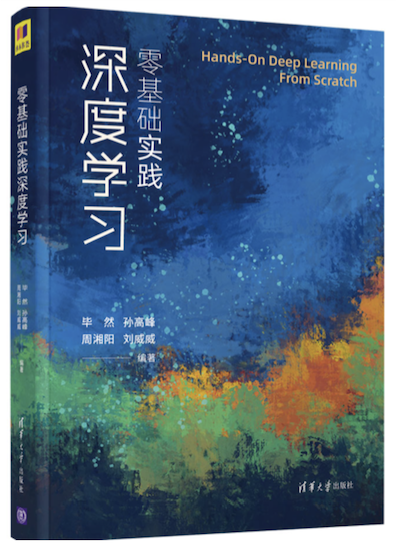#### 2.2.2. 模型结构

EAST的网络结构总共包含三个部分：Feature extractor stem（特征提取分支）, Feature-merging branch（特征合并分支） 以及 Output layer（输出层），网络结构如 图2 所示：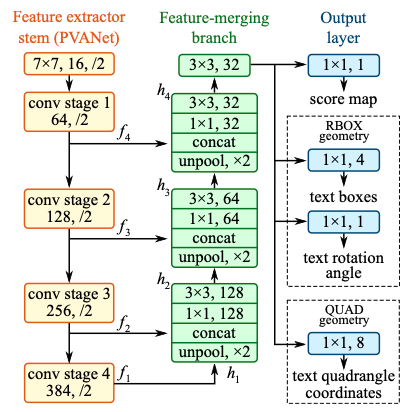- 特征提取分支

 - 特征合并分支

• 上一步提取的feature map f1被最先送入unpool层(将原特征图放大２倍)；
• 然后与前一层的feature map f2进行拼接；
• 接着依次送入卷积核大小为1×1和3×3的卷积层，核数通道数随着层递减，依次为128，64，32；
• 重复上面三个步骤２次；
• 最后将经过一个卷积核大小为3×3，核数通道数为32个的卷积层；

 - 输出层

• RBOX：主要用来预测旋转矩形的文本，包含文本得分和文本形状(AABB boundingbox 和rotate angle)，一共有６个输出，这里AABB分别表示相对于top，right，bottom，left的偏移；
• QUAD：用来预测不规则四边形的文本，包含文本得分和文本形状(８个相对于corner vertices的偏移)，一共有９个输出，其中QUAD有８个，分别为 $(x_{i},y_{i}), i\in[1,2,3,4]$。

#### 2.2.3. 模型loss

EAST损失函数由两部分组成，具体公式如下：

$$L=L_{s}+λ_{g}L_{g}$$

 - 分数图损失

$$L_{s} = -\beta Y^{*}log(\hat{Y})-(1-\beta)（1-Y^*）log(1-\hat{Y})$$

 - 几何损失

• RBOX：IOU损失

$$L_{AABB} = -log\frac{\hat{R}\cap R^*}{\hat{R}\cup R^*}$$

$$L_g=L_{AABB} + \lambda L_\theta$$

$$L_g = \min\limits_{\tilde{Q}\in P_{Q^*}} \sum\limits_{c_i \in C_Q, \tilde c_i \in C_\tilde Q}\frac{smoothed_{L_1}(c_i, \tilde{c}_i)}{8*N_{Q^*}}$$

$$N_{Q^*} = \min\limits_{i=1}^{4} D(p_i, p_{(i mode 4)+1})$$

• 可以检测多方向的文本

• 不能检测弯曲文本

### 2.3. DBNet

DBNe（Real-time Scene Text Detection with Differentiable Binarization）对这个流程进行了优化，对每个像素点进行自适应二值化，二值化的阈值由网络学习得到，彻底将二值化这一步骤加入到网络里一起训练，这样最终的输出图对于阈值就会非常鲁棒。

#### 2.3.1. 模型输入标签

DB网络中，网络的输出为3个部分：概率图、阈值图和近似二值图：

• 概率图：图中每个像素点的值为该位置属于文本区域的概率。
• 阈值图：图中每个像素点的值为该位置的二值化阈值。
• 二值图：由概率图和阈值图通过DB算法计算得到，图中像素的值为0或1。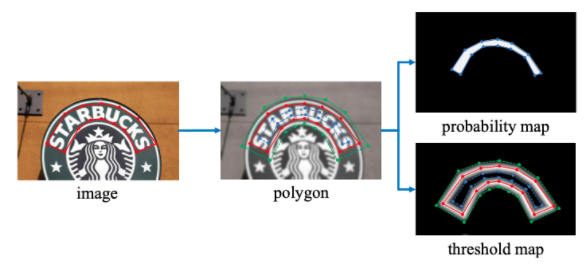$$D = \frac{A(1-r^2)}{L}$$

#### 2.3.2. 模型介绍

DB文本检测模型使用了标准的FPN结构，网络结构如 图2 所示。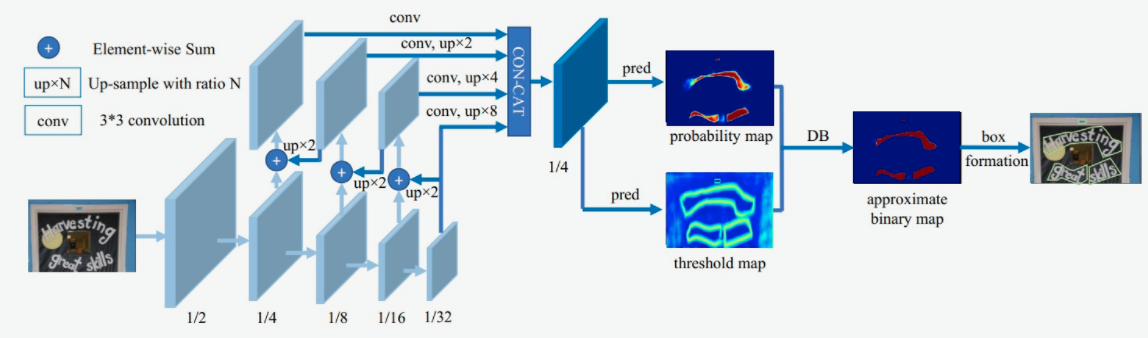- 第一模块：使用CNN网络，对输入图像提取特征，得到特征图，本实验使用的骨干网络是轻量化网络MobileNetv3，同时使用了FPN结构，获取多尺度的特征，在本实验中，我们提取4个不同尺度下的特征图做拼接。

 - 第二模块：使用一个卷积层和两个转置卷积层的结构获取预测的概率图和阈值图；

 - 第三模块：使用DB方法获取近似二值图。

$$B_{i,j} = 1,if P_{i,j} >= t, otherwise \ 0$$

$$\hat{B} = \frac{1}{1 + e^{-k(P_{i,j}-T_{i,j})}}$$

$$l_+ = -log(\frac{1}{1 + e^{-k(P_{i,j}-T_{i,j})}})$$

$$l_- = -log(1-\frac{1}{1 + e^{-k(P_{i,j}-T_{i,j})}})$$

$$\frac{\delta{l_+}}{\delta{x}} = -kf(x)e^{-kx}$$

$$\frac{\delta{l_-}}{\delta{x}} = -kf(x)$$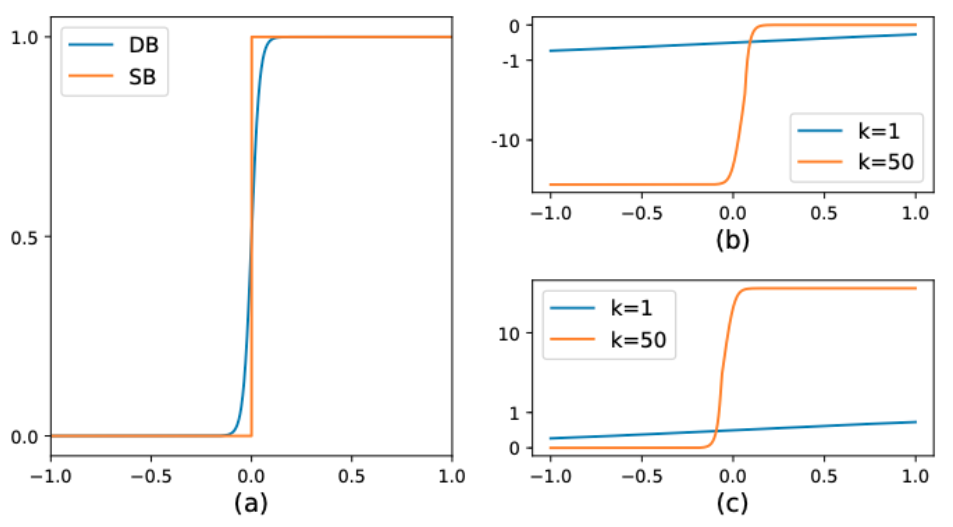#### 2.3.3. 模型loss

$$L = L_b + \alpha \times L_s + \beta \times L_t$$

 - 首先是Dice Loss，Dice Loss是比较预测结果跟标签之间的相似度，常用于二值图像分割。

$dice\_loss = 1 - \frac{2 \times intersection\_area}{total\_area}$

 - 其次是MaskL1 Loss，是计算预测值和标签间的$L_1$距离

 - 最后是Balance Loss，是带OHEM的Dice Loss，目的是为了改善正负样本不均衡的问题。OHEM为一种特殊的自动采样方式，可以自动的选择难样本进行loss的计算，从而提升模型的训练效果。

#### 2.3.4. 模型优缺点

• 可以同时检测水平、多方向和弯曲文字；
• 在性能和速度上都获取不错的效果。

## 3. OCR 识别方法

### 3.1. CRNN

#### 3.1.2. 模型结构

CRNN的主要结构包括基于CNN的图像特征提取模块以及基于多层双向LSTM的文字序列特征提取模块。CRNN的网络结构如 图1 所示：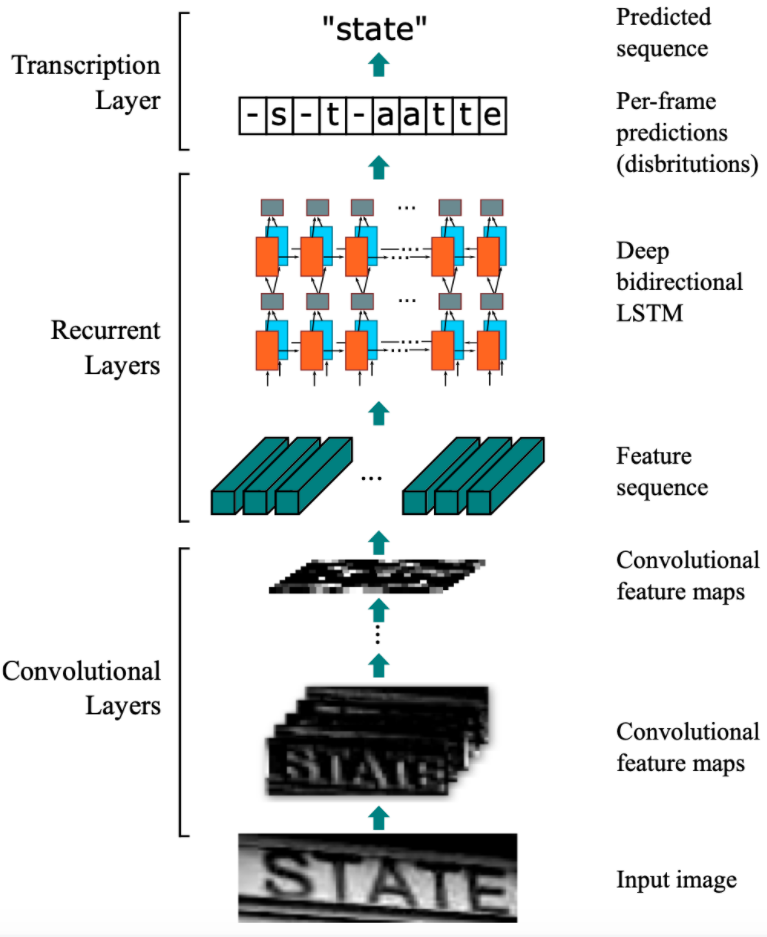- 第一模块：使用CNN网络，对输入图像进行特征提取，从而得到特征图。本实验使用的CNN网络同样是轻量化网络MobileNetv3，其中输入图像的高度统一设置为32，宽度可以为任意长度，经过CNN网络后，特征图的高度缩放为1；

 - 第二模块：Im2Seq，将CNN获取的特征图变换为RNN需要的特征向量序列的形状；

 - 第三模块：使用双向LSTM（BiLSTM）对特征序列进行预测，学习序列中的每个特征向量并输出预测标签分布。这里其实相当于把特征向量的宽度视为LSTM中的时间维度；

 - 第四模块：使用全连接层获取模型的预测结果；

 - 第五模块：CTC转录层，解码模型输出的预测结果，得到最终输出。

#### 3.1.4. 模型优缺点

• 可以进行端到端的训练；
• 可以进行不定长文本的识别；
• 模型简单，效果好。

• 受CTC算法对速度的要求，输出长度受到限制，识别文本不能太长。
Last modification：September 18th, 2021 at 02:21 pm